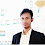# Important objective questions

Important objective questions for higher secondary level and Diploma level students :

1. What is the cgs unit of electric

charge?

1. coulomb

2. stat coulomb

3. mili coulomb

4. ampere

2. Find the correct dimensional

Formula of Electric charge?

1. [ M1 L1 T-1 ]

2. [ Mo Lo To C1 ]

3. [L1 T-1 A-1 ]

4. [ A1 T1 ]

3. Minimum charge that can

exist is

1. 1 C

2. 6.25 × 1018 C

3. 1.6 × 10-19  C

4. 0.001 C

4. How many electons are there

in 1 C ?

1. 1 electron

2. 1.16 × 10-19 electrons

3. 6.28 × 1018 electrons

4. Can't be found

5. What is the unit of Electric

field ?

1. V / m

2. Nm2 / C

3. volt (V)

4. Nm2 / C2

6. Find the correct dimensional

Formula for Electric field.

1. [ M1 L1 T-1 A-1 ]

2. [ M1 L1 T-1 ]

3. [ M1 L1 T-3 A-1]

4. [ Mo L1 T-1 A-1]

7. Find the charge on a body

having 2 × 107 electrons.

1. 3.2 × 10-16 C

2. 1.6 × 10-16 C

3. 3.2 × 10-12 C

4. 1.6 × 1012  C

8. Two unit charges are placed

at unit distance, what would

be the force of interaction

between them ?

1. 1 N

2. 2.56 × 10-38 N

3. 9 × 109 N

4. Can't be found

9.Write the dimensional

Formula for absolute

electrical Permittivity of

medium.

1. [ M1 L1 T-3 A-1 ]

2. [ M1 L3 T-1 A-3 ]

3. [ M-1 L-2 T-4 A-3 ]

4. [ M-1 L-3 T4 A2 ]

10. What is the value of

absolute electrical

permittivity of free space ?

1. 9 × 109 Nm2 / C2

2. 8.85 × 10-12 Nm2 / C2

3. 9 × 10C2 N-1m-2

4. 8.85 × 10-12 C2 N-1m-2

11. if a body gives out 109

electrons per second, how

much time is required to get

a total charge of 1 C from

it?

1. 6.25 × 1013 s

2. 198 year

3. 6.25 × 1011 s

4. 158 year

12. What is the dimensional

formula of electric flux?

1. [ M1 L3 T-3 A-1 ]

2. [ M1 L3 T-1 A-3 ]

3. [ M1 L2 T-1 A-1 ]

4. [ M2 L3 T-2 A-3 ]

1.Ans1.-coulomb

1.Stat coulomb

2.Ans3.-1.6×10^-19 C

3.Ans5.- V/m or N/C

4.And.- [M^1L^1T^-3A^-1]

1.5.Ans.7- 3.2×10^-12 C

6.Ans.2-[M^0L^0T^1A^1]

7.8.10.
Ans- D

9.11.Ans-198 year

1.2.Thanks Sir

10.11.4.ans - 6.25×10^18 electron